## Code and Output

### Code 13.2.1:

``````format long;
Timenow = GetSecs;``````

### Output 13.2.1:

``````Timenow =
8.007085074686600e+04``````

### Code 13.2.2:

``ScreenTest``

### Output 13.2.2:

``````***** ScreenTest: Testing Screen 0 *****

PTB-INFO: This is Psychtoolbox-3 for Apple OS X, under Matlab 64-Bit (Version 3.0.11 - Build date: Jul 23 2013).
PTB-INFO: Type 'PsychtoolboxVersion' for more detailed version information.
...``````

### Code 13.3.1:

``````mywindow = Screen('OpenWindow',0);
Screen('DrawText', mywindow, 'Hello World!',200,100,[0,0,0]);
Screen('Flip',mywindow);
WaitSecs(1);
sca``````

### Output 13.3.1:

``Hello World!``

### Code 13.4.1:

``help Screen``

### Code 13.4.2:

``Screen DrawText?``

### Output 13.4.2:

``````Usage:

[newX,newY]=Screen('DrawText', windowPtr, text [,x] [,y] [,color] [,backgroundColor] [,yPositionIsBaseline] [,swapTextDirection]);

Draw text. "text" may include Unicode characters (e.g. Chinese).A standard MATLAB/Octave character text string is interpreted according to Screen's current character encoding setting
...``````

### Code 13.5.1:

``````Screen('Preference', 'VisualDebugLevel', 1);
window = Screen('OpenWindow',0);
Screen('TextSize',window, 50);
Screen('TextFont',window, 'Times');
Screen('DrawText',window, 'Hello World!',100,100,[0,0,0]);
Screen('Flip',window);
WaitSecs(1)
sca``````

### Code 13.5.2:

``````window = Screen('OpenWindow',0,[255,0,0]);
Screen('Flip',window);
WaitSecs(1)
sca``````

### Code 13.6.1:

``````Screen('Preference', 'VisualDebugLevel', 1);
mywindow = Screen('OpenWindow', 0);
Screen('TextSize',mywindow, 50);
Screen('TextFont',mywindow, 'Times');
Screen('DrawText',mywindow, 'Hello World!', 100,100,[0,0,0]);
Screen('FrameOval',mywindow, [200 0 200], [75 50 225 200],5);
Screen('Flip',mywindow);
WaitSecs(1);
Screen('Flip',mywindow);
WaitSecs(1);
sca``````

### Output 13.6.1: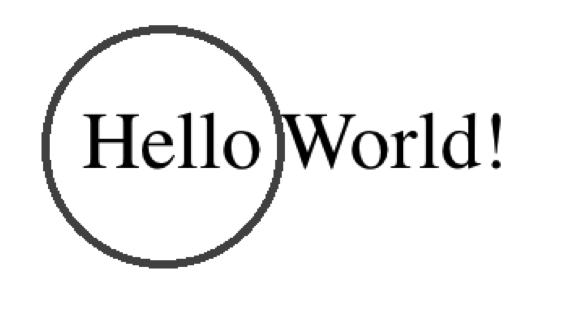### Code 13.7.1:

``````imagedata = imread('lab_photo.jpg');
window = Screen('OpenWindow',0);
TexturePointer = Screen('MakeTexture', window, imagedata);
clear imagedata;
Screen('DrawTexture', window, TexturePointer);
Screen('Flip', window);
WaitSecs(2);
sca``````

### Output 13.7.1: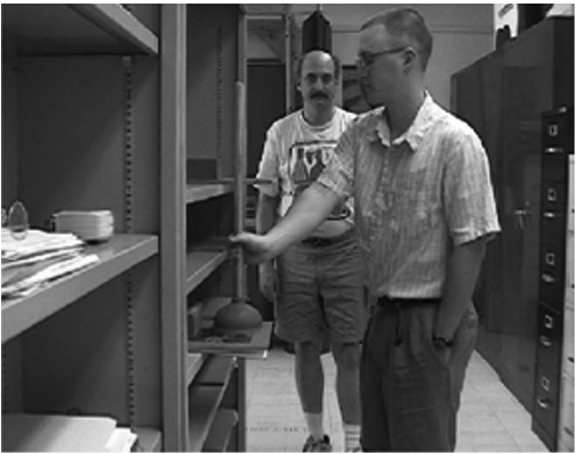### Code 13.8.1:

``````Screen('Preference', 'VisualDebugLevel', 1);
window = Screen('OpenWindow',0);
Screen('FillOval',window,[0,200,200],[200,200,250,250]);
onsetTime1 = Screen('Flip',window);
WaitSecs(1);
Screen('FillOval',window,[0,200,200],[200,300,250,350]);
onsetTime2 = Screen('Flip',window);
WaitSecs(2);
sca``````

### Code 13.8.2:

``onsetTime2-onsetTime1``

### Output 13.8.2:

``````ans =
1.0188``````

### Code 13.8.3:

``````Screen('Preference', 'VisualDebugLevel', 1);
window = Screen('OpenWindow',0);
halfFlip = Screen('GetFlipInterval', window)/2;
Screen('FillOval',window,[0,200,200],[200,200,250,250]);
onsetTime1 = Screen('Flip',window);
Screen('FillOval',window,[0,200,200],[200,300,250,350]);
onsetTime2 = Screen('Flip',window,onsetTime1 + 1.0 - halfFlip);
WaitSecs(2);
myduration = onsetTime2 - onsetTime1
sca``````

### Output 13.8.3:

``````myduration =
0.9997``````

### Code 13.9.1:

``>>x = KbCheck(-1)``

### Output 13.9.1:

``````x =
1``````

### Code 13.9.2:

``[KeyIsDown secs keyCode] = KbCheck(-1);``

### Code 13.9.3:

``KbName(keyCode)``

### Output 13.9.3:

``````ans =
Return``````

### Code 13.9.4:

``KbName('UnifyKeyNames')``

### Code 13.10.1:

``````while(KbCheck(-1))
end

waitTime = 5;
nowTime = GetSecs;
endTime = nowTime + waitTime;
keyDown = 0;

ListenChar(2);
while(keyDown ==0) & (nowTime < endTime)
keyDown = KbCheck(-1);
nowTime = GetSecs;
end

if(keyDown ==1)
'A key was pressed'
else
'Ran out of time'
end
ListenChar(0);
sca``````

### Code 13.11.1:

``````KbName('UnifyKeyNames');
Chars = 0;
maxChars = 5;
Response = [];

[KeyIsDown secs keyCode] = KbCheck(-1);

ListenChar(2)
while Chars < maxChars
lastkeyCode = keyCode;
[KeyIsDown secs keyCode] = KbCheck(-1);

difference = keyCode - lastkeyCode;
keys = find(difference == 1);

for(i = 1: length(keys))
if(Chars < 5)
Chars = Chars + 1;
Response = [Response KbName(keys(i))];
end
end
end
ListenChar(0)
fprintf('The typed response was \n%s\n',Response)``````

### Output 13.11.1:

``````The typed response was
abspacecd``````

### Output 13.11.2:

``````The typed response was
op[{]}\|``````

### Code 13.12.1:

``````% Part One: Initialization
Screen('Preference', 'VisualDebugLevel', 1);
window = Screen('OpenWindow',0);
Screen('TextSize',window,24);
Screen('TextFont',window,'Times');
circlecenter = 400;
textX = 200;
textY = 200 ;
Cursorsize= 6;  %how big our mouse cursor will be
mousedata = zeros(10000,2);  %used to store mouse data points
sample = 0;
%move the mouse to a specific spot
HideCursor; %hide the existing mouse cursor

% Part Two: Mousewait
buttons = 1;
while any(buttons)
[Mousex,Mousey,buttons] = GetMouse(window);
end

% Part Three: Collect Data
DesiredSampleRate = 10          %Number of samples per second
clear sampletime;
begintime = GetSecs;
nextsampletime = begintime;
while buttons(1) ==0
sample = sample + 1;
xlocation = 0;
Screen('DrawText',window',...
'Trace the Circle clockwise, then click left button',...
textX,textY,[0, 0, 0 ]);
Screen('FrameOval', window , [0, 0, 0 ],[lowerbound ...
lowerbound upperbound upperbound],4);
Screen('FrameOval', window , [0, 0, 0] ,[Mousex-Cursorsize,...
Mousey-Cursorsize,Mousex+Cursorsize,Mousey+Cursorsize],3);
Screen('Flip',window);
[Mousex,Mousey,buttons] = GetMouse(window);
mousedata(sample,1) = Mousex;
mousedata(sample,2) = Mousey;
sampletime(sample) = GetSecs;
nextsampletime = nextsampletime + 1/DesiredSampleRate;
while GetSecs < nextsampletime
end
end

% Part Four: Cleanup
endtime = GetSecs;
ElapsedTime = endtime - begintime
NumberOfSamples = sample
ActualSampleRate = 1/(ElapsedTime / NumberOfSamples)
mousedata = mousedata(1:sample,1:2);
ShowCursor;
sca
size(mousedata)
clf;
plot(mousedata(:,1), mousedata(:,2));
set(gca,'YDir','reverse');
axis equal
shg``````

### Output 13.12.1a: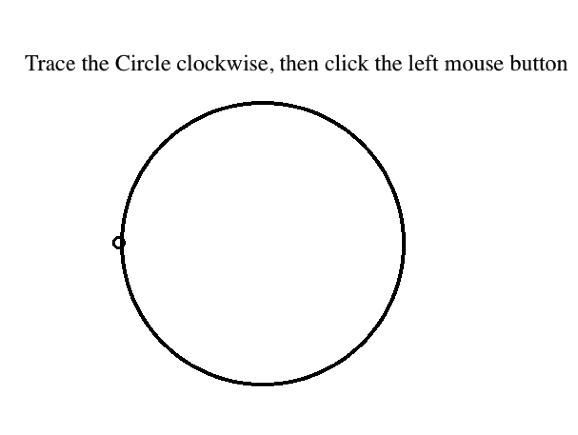### Output 13.12.1b: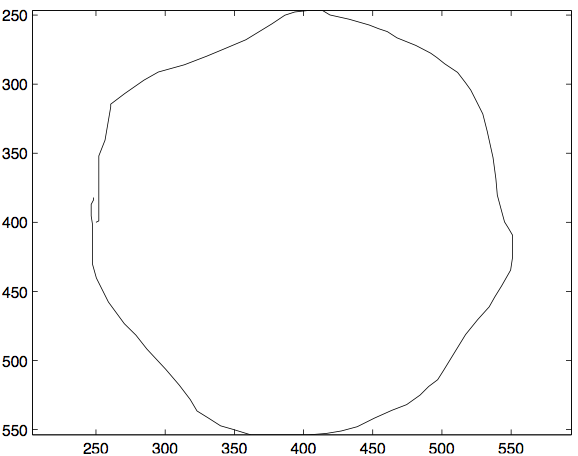``````DesiredSampleRate =
10
ElapsedTime =
8.7009
NumberOfSamples =
87
ActualSampleRate =
9.9990``````

### Code 13.12.2:

``````...
% Part Three: Collect Data
DesiredSampleRate = 100         % Number of samples per second
clear sampletime;
...``````

### Output 13.12.1c:

``````DesiredSampleRate =
100
ElapsedTime =
4.5254
NumberOfSamples =
271
ActualSampleRate =
59.8843``````

### Code 13.13.1:

``````% Part One:  Initialization
Screen('Preference', 'VisualDebugLevel', 1);
[window  Scrnsize]= Screen('OpenWindow',0);
fliptime = Screen('GetFlipInterval', window);
centerX = Scrnsize(3)/2;
centerY = Scrnsize(4)/2;
coherence = .5;
numdots = 1000;
dotspeed = 2;
dotpos =  zeros(2,numdots);
dotdir = zeros(1,numdots);
boxsize = 600;
trialduration = 3.0;
uniformdirection = randi(4);  %1 = down, 2 = left, 3 = up, 4 = right
for(dot = 1:numdots)
dotpos(1,dot) = randi(boxsize); %Horizontal starting position
dotpos(2,dot) = randi(boxsize); %Vertical starting position
if(dot < ceil(numdots*coherence))
dotdir(1,dot) = uniformdirection*pi/2;
else
dotdir(1,dot) = rand*2*pi;
end
end

% Part Two: Animation
starttime = GetSecs;
timestamps = zeros(trialduration/fliptime,1);
counter = 0;
while(GetSecs-starttime < trialduration)
for(dot = 1:numdots)
dotpos(1,dot) =  dotpos(1,dot) + cos(dotdir(dot))*dotspeed;
dotpos(2,dot) =  dotpos(2,dot) + sin(dotdir(dot))*dotspeed;

if(dotpos(1,dot) > boxsize)
dotpos(1,dot)=  dotpos(1,dot)- boxsize;
end
if(dotpos(2,dot) > boxsize)
dotpos(2,dot) =  dotpos(2,dot)- boxsize;
end
if(dotpos(1,dot) < 1)
dotpos(1,dot)=  dotpos(1,dot)+ boxsize;
end
if(dotpos(2,dot) < 1)
dotpos(2,dot)=  dotpos(2,dot)+ boxsize;
end
end
counter = counter + 1;
Screen('Drawdots',window,dotpos,2,[255,0,0],[centerX-...
boxsize/2, centerY-boxsize/2],1);
timestamps(counter) = Screen('Flip',window);
end

% Part Three: User Response
sca
Response = input('Which direction did most of the dots move?\n 1= down, 2 = left, 3 = up, 4 = right:  ');
if(Response == uniformdirection)
'You are correct'
else
end``````

### Output 13.13.1: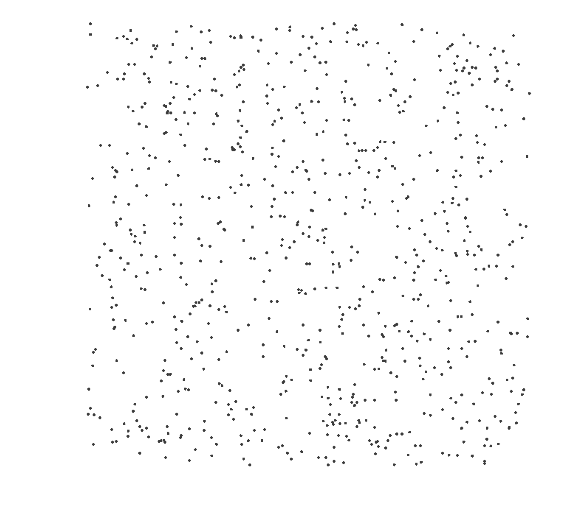### Code 13.14.1:

``````Screen('Preference', 'VisualDebugLevel', 1);
window = Screen('OpenWindow',0,[150,150,150]);
Screen('Blendfunction', window, GL_SRC_ALPHA, ...
GL_ONE_MINUS_SRC_ALPHA);
Screen('FillOval',window,[0,0,255,75],[200,200,350,350]);
Screen('FillOval',window,[0,255,0,75],[300,200,450,350]);
Screen('FillOval',window,[255,0,0,75],[250,250,400,400]);
Screen('Flip',window);
KbPressWait(-1)
sca``````

### Code 13.14.2:

``AlphaImageDemo``

### Code 13.15.1:

``````% Part One:  Initialization
Screen('Preference', 'VisualDebugLevel', 1);
sinit = input('Subject''s initials: ','s');
outfilename = ['SimonDataPTB_' sinit];
[window  Scrnsize]= Screen('OpenWindow',0);
halfFlip = Screen('GetFlipInterval', window)/2;
KbName('UnifyKeyNames');
centerX = Scrnsize(3)/2;
centerY = Scrnsize(4)/2;
Screen('TextFont',window, 'Arial');
Screen('TextSize',window, 72);
timeout = 2;
[ttype(1:4).side] = deal('L','R','L','R');
[ttype(1:4).stim] = deal('L','L','R','R');
[ttype(1:4).comp] = deal('C','I','I','C');
% Prepare data fields for each type of trial.
[ttype(1:4).RT] = deal([]);
[ttype(1:4).error] = deal(0);
%get the size of the fixation cross
[bounds] = Screen('TextBounds', window, '+');
fixSizeX = bounds(3)/2;
%get the size of the stimuli
[bounds] = Screen('TextBounds', window, 'L');
stimSizeX = bounds(3)/2;
leftStimX = centerX-400-stimSizeX;
rightStimX = centerX+400-stimSizeX;
ListenChar(2);

% Part Two:  Data Collection
HideCursor
for blocknumber = 1:8
for typenum = randperm(4);
WaitSecs(2);   %pause at start of trial, then show fixation
Screen('DrawText', window , '+',centerX-fixSizeX ,...
centerY,[0,0,0]);
onsetTime1 = Screen('Flip',window);
%Draw the fixation cross
Screen('DrawText', window , '+',centerX-fixSizeX ,...
centerY,[0,0,0]);
%Show the stimulus on the left or right side
if ttype(typenum).side == 'L'
Screen('DrawText', window , ttype(typenum).stim,...
leftStimX,centerY,[0,0,0]);
else
Screen('DrawText', window , ttype(typenum).stim,...
rightStimX,centerY,[0,0,0]);
end
Starttime  = Screen('Flip',window,onsetTime1 + 1.0 ...
- halfFlip);
Nowtime = Starttime;
responseGiven = 0;
response = 0;
%collect a response with a timeout
while(Nowtime < Starttime + timeout & responseGiven == 0)
%Check for a response
[keyDown secs keyCode] = KbCheck(-1);
if(keyDown)
responseGiven = 1;
response = KbName(keyCode);
end
Nowtime = GetSecs;       % check the current time
end
thisRT = secs-Starttime;   %compute the reaction time

if(response(1)=='a')   %convert the response into L or R
thisResp = 'L';
elseif(response(1) == ';')
thisResp = 'R';
else
thisResp = 'X';
end
if ttype(typenum).stim == thisResp
ttype(typenum).RT = [ttype(typenum).RT thisRT];
else
ttype(typenum).error = ttype(typenum).error + 1;
Beeper;
end
Screen('Flip',window);
end
end

% Part Three:  Cleanup and File save
ShowCursor
ListenChar(0);
sca
save(outfilename,'ttype');``````

### Output 13.15.1: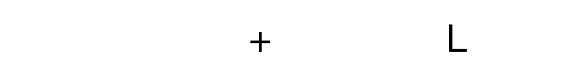### Code 13.16.1:

``help PsychDemos``

### Code 13.16.2:

``edit DriftDemo``

## Solutions

``````% Solutions_Chapter_13

% Solutions for selected problems from MATLAB for Behavioral Scientists,
% Second Edition (D. A. Rosenbaum, J. Vaughan, & B. Wyble),
% (c) 2015, Taylor & Francis

% To generate the solution for one problem, copy and run the code for that
% problem in a file or paste it into the Command window. Show the Command
% window to see the results.

% Because the program's interaction with the user is very dynamic, the
% web page cannot show the Command window or Graphic output for the
% problems of Chapter 13. The best way to explore Psychtoolbox is to run
% each of the examples separately.

% You can download a folder with this and two other files needed to run the

function main % Problems in this chapter will have nested or local
% functions so the Problem 13 file must itself be a function
close all
clc
commandwindow
% Screen('Preference', 'SkipSyncTests', 1); % If you need to!
Solution_13_18_1 %Run each of the problem functions in turn.
Solution_13_18_2
Solution_13_18_3
Solution_13_18_4
Solution_13_18_6
Solution_13_18_7
Solution_13_18_8
Solution_13_18_10
Solution_13_18_11

shg
end % function main

% Problem 13.18.1
% The DrawTexture operation can place a texture multiple times.
% Modify the code of 13.7.1 to place three copies of the same
% image at different locations on the screen simultaneously at
% three different locations and at three different sizes. To
% resize the image, you will need to know its original dimensions
% which you can get from the imagedata variable before its cleared.
% You will need to use the parameters of DrawTexture named sourceRect
% Each of these is a vector containing four numbers that specify the
% corners of the image, just like the location parameter to FrameOval
% in Code 13.6.1.  The variable sourceRect specifies the part of the
% texture you are copying from and the destinationRect specifies the
% destination you are copying too on the screen. If the destination
% rectangle is a different size than the source rectangle, the
% texture will be grown or shrunk automatically as appropriate so it fits.

function Solution_13_18_1
try
catch
sprintf('This function requires the file lab_photo.jpg to work')
return
end
window = Screen('OpenWindow',0);
imagesize = size(imagedata);
TexturePointer = Screen('MakeTexture', window, imagedata);
clear imagedata;
Screen('DrawTexture', window, TexturePointer,[0 0 imagesize(2) imagesize(1)],...
[1 1 imagesize(2)+100 imagesize(1)+1]);
Screen('DrawTexture', window, TexturePointer,[0 0 imagesize(2),imagesize(1)],...
[1 500 imagesize(2)+1 imagesize(1)+600]);
Screen('DrawTexture', window, TexturePointer,[0 0 imagesize(2),imagesize(1)],...
[900 600 1050 670]);
Screen('Flip', window);
WaitSecs(2);
sca
end

% Problem 13.18.2
% Modify your solution to 13.18.1 by adding a for loop so that 10
% copies of the texture appear on the screen in sequence, each one
% rotated by 36 degrees relative to the previous one and on the
% screen for precisely 300 milliseconds.

function Solution_13_18_2
try
catch
sprintf('This function requires the file lab_photo.jpg to work')
return
end

window = Screen('OpenWindow',0);
halfFlip = Screen('GetFlipInterval', window)/2;
SOA = .3;  %time between image changes
imagesize = size(imagedata);
TexturePointer = Screen('MakeTexture', window, imagedata);
clear imagedata;
lasttime = Screen('Flip', window);
for(pic = 1:10)  %draw the image 10 times
Screen('DrawTexture', window, TexturePointer,[0 0 imagesize(2),...
imagesize(1)],[500 300 imagesize(2)+300 imagesize(1)+100],36*pic);
%the time of each flip is the previous flip time + the SOA variable
lasttime = Screen('Flip', window,lasttime + SOA-halfFlip);
end
sca
end

% Problem 13.18.3
% Apparent motion occurs when a stimulus appears to move
% between two locations even though the stimulus is shown at one
% location, A, then at another location, B, then at A again, then
% at B again, and so on. Create a Psychtoolbox program to draw a
% circle that alternates between two locations repeatedly until you
% press any key, at which point the program exits. Make the circles
% 20 pixels in diameter and have the program wait 150 milliseconds
% between jumps. Use the method illustrated in Code 13.8.3 to ensure
% that your stimulus timing is precise. Modify the number of pixels
% between the two presentations of the circle to find the critical
% distance at which the circle appears to move back and forth, rather
% than blink on and off. You will then have created an apparent
% motion demonstration.

function Solution_13_18_3

window = Screen('OpenWindow',0);
SOA = .15;  %temporal lag between the two dots
offset = 100;  %spatial separation between the two dots in pixels
diameter=  30;  %size of the dots in pixels
ListenChar(2);
halfFlip = Screen('GetFlipInterval', window)/2;

while(KbCheck(-1) ==1)  %wait for no keys to be pressed
end
HideCursor;
kb =0;
time1 = GetSecs;
while (kb == 0)  %loop until any key is pressed
%draw the first dot
Screen('FillOval', window , [0, 200, 200 ,100] ,...
[200, 200, 200 + diameter, 200 + diameter]);
time2 =  Screen('Flip',window,time1+SOA-halfFlip);
kb1 = KbCheck(-1);
%draw the second dot
Screen('FillOval', window , [0, 200, 200 ,100] ,[200+offset,...
200, 200+ offset + diameter, 200 + diameter]);
time1 = Screen('Flip',window,time2+SOA-halfFlip);

%see if there was a key pressed during this iteration of the loop
kb2 = KbCheck(-1);
if(kb1 | kb2)
kb = 1;
end

end
ListenChar(0);
ShowCursor;
sca
end

% Problem 13.18.4
% Now modify your solution to 13.18.4 so one presentation of
% the circle is presented at a fixed location and the other circle
% is presented at the current mouse position. This should allow you
% to control the separation of the circles with great precision.
% Now use your program to find the largest separation at which two
% separate dots appear as one dot moving back and forth, by adjusting
% the separation using the mouse.

% Now modify your program to use DrawText to display the number of
% pixels between the centers of the two dots near the bottom of the
% screen.

% Now use your program to discover
% the maximum separation distance, measured in screen pixels, at which
% the apparent motion illusion can occur. Remember to keep your eyes a
% fixed distance from the monitor while viewing the stimuli in the
% experimental display. Measure this distance because you will need
% it for the next problem.

function Solution_13_18_4
window = Screen('OpenWindow',0);
halfFlip = Screen('GetFlipInterval', window)/2;

Screen(window, 'TextSize',50);
SOA = .150;
diameter=  30;
ListenChar(2);
offset = 100;
while(KbCheck(-1) ==1)
end

HideCursor;
kb =0;
time1 = GetSecs;
[Mousex,Mousey,buttons] = GetMouse(window);
printstring = '0';

while (kb == 0)
% draw fixed dot
Screen('FillOval', window , [0, 200, 200 ,100] ,...
[200, 200, 200 + diameter, 200 + diameter]);
Screen('DrawText', window , printstring,200,400);
time2 =  Screen('Flip',window,time1+SOA-halfFlip);
kb1 = KbCheck(-1);

% Get the mouse location
[Mousex,Mousey,buttons] = GetMouse(window);
distance = sqrt((Mousex-200)^2+(Mousey-200)^2); % distance between mouse and dot #1
printstring = sprintf('%d',ceil(distance));
Screen('DrawText', window , printstring,200,400);  % write that distance on the screen

% draw the mouse-locked dot
Screen('FillOval', window , [0, 200, 200 ,100] ,...
[Mousex, Mousey, Mousex + diameter, Mousey + diameter]);
time1 = Screen('Flip',window,time2+SOA -halfFlip);

kb2 = KbCheck(-1);  % check if a key was pressed at either of the KbChecks
if(kb1 | kb2)
kb = 1;
end
end
ListenChar(0);
ShowCursor;
sca
end

% Problem 13.18.6

% Modify Code 13.10.1 so it reports the name of the key that was
% pressed and the reaction time relative to the start of the while loop.

function Solution_13_18_6
while(KbCheck(-1))  %wait until no keys are pressed to start
end
sprintf('Press any key')
waitTime = 5;
nowTime = GetSecs;
endTime = nowTime + waitTime;  %set the time to end
keyDown = 0;
KbName('UnifyKeyNames');
ListenChar(2);
startTime = GetSecs;
%loop until a key is pressed or the deadline expires
while(keyDown ==0) & (nowTime < endTime)
[keyDown keyTime keyCode]  = KbCheck(-1);
nowTime = GetSecs;
end
keyname = KbName(keyCode);
if(keyDown ==1)
sprintf('Key pressed was %s after %g seconds',keyname,keyTime-startTime)
else
'Ran out of time'
end
ListenChar(0);
sca
end

% Problem 13.18.7
% Modify the code of 13.12.1 so that the while loop ends when the
% user has completed the circle, rather than when the mouse button is
% clicked. To do this, you will need to draw a marker on the circle to
% remind the user of the point they started at. You can use many
% Psychtoolbox functions to do this, but we suggest DrawLine. You will
% also need to compute the distance between the mouse position and this
% starting point, and end the main while loop when that distance is
% sufficiently short.

function Solution_13_18_7
% Part One: Initialization
Screen('Preference', 'VisualDebugLevel', 1);
window = Screen('OpenWindow',0);
Screen('TextSize',window,24);
Screen('TextFont',window,'Times');
circlecenter = 400;
textX = 200;
textY = 200 ;
Cursorsize= 6;  % how big our mouse cursor will be
mousedata = zeros(10000,2);  % used to store mouse data points
sample = 0;
% move the mouse to a specific spot
HideCursor; % hide the existing mouse cursor
movedaway = 0;  % have we moved far enough away from start point?
criticaldistance = 10;  %number of pixels defining "far enough"
movedback = 0;

% Part Two: Mousewait
buttons = 1;
while any(buttons)
[Mousex,Mousey,buttons] = GetMouse(window);
end

% Part Three: Collect Data
DesiredSampleRate = 10;          % Number of samples per second
clear sampletime;
begintime = GetSecs;
nextsampletime = begintime;
%Loop until the mouse cursor has moved away from the start and then back
while movedback == 0;
sample = sample + 1;
xlocation = 0;
% draw instructions
Screen('DrawText',window,...
'Trace the Circle clockwise back to the starting position',...
textX,textY,[0, 0, 0 ]);
% draw the big circle
Screen('FrameOval', window , [0, 0, 0 ],[lowerbound ...
lowerbound upperbound upperbound],4);
% draw the mouse cursor
Screen('FrameOval', window, [0, 0, 0] ,[Mousex-Cursorsize,...
Mousey-Cursorsize,Mousex+Cursorsize,Mousey+Cursorsize],3);
Screen('DrawLine',window,[0,0,0],...
lowerbound-8,circlecenter,lowerbound+8,circlecenter,5);

Screen('Flip',window);
% get the new position of the mouse and added it to mousedata
[Mousex,Mousey,buttons] = GetMouse(window);
mousedata(sample,1) = Mousex;
mousedata(sample,2) = Mousey;
sampletime(sample) = GetSecs;

% check the distance between the mouse cursor and the starting point
distance = sqrt((Mousex- lowerbound)^2+ (Mousey- circlecenter)^2);
if(distance  > criticaldistance)
% enable this flag once the cursor has moved sufficiently far from starting point
movedaway = 1;
end
if(distance  < criticaldistance & movedaway)
% enable this flag once the cursor has moved back to the starting point
movedback = 1;
end

nextsampletime = nextsampletime + 1/DesiredSampleRate;
while GetSecs < nextsampletime
end
end

% Part Four: Cleanup
endtime = GetSecs;
ElapsedTime = endtime - begintime
NumberOfSamples = sample
ActualSampleRate = 1/(ElapsedTime / NumberOfSamples)
mousedata = mousedata(1:sample,1:2);
ShowCursor;
sca
size(mousedata)
clf;
plot(mousedata(:,1), mousedata(:,2));
set(gca,'YDir','reverse');
axis equal
shg

end

% Problem 13.18.8
% Create a program to determine the minimum duration that a stimulus
% has to be on the screen to be seen if it is followed by another stimulus.
% Your program should specify a list of four-letter words in a cell array.
% It should then pick one of the words randomly and display it in the center
% of the screen, in uppercase letters. After a number of milliseconds has
% elapsed, replace the word with the string ?####? at exactly the same
% spatial position as the word, to serve as a mask. Leave this mask on the
% screen for exactly 1 second and then exit the program. Now, determine the
% smallest duration at which you can still determine which of the 4 words was
% presented. Ensure that your program has accurate timing by using the techniques
% shown in Code 13.8.3.

function Solution_13_18_8
Screen('Preference', 'VisualDebugLevel', 1);
window = Screen('OpenWindow',0);
halfFlip = Screen('GetFlipInterval', window)/2;
wordlist = {' HEAR',' FROM',' DRAB',' DROP'};
trials = 10;
textX = 200;
textY = 200;
maskdelay = .05;   % how long is the word on the screen before the mask onsets?
maskduration = 1;  % how long is the mask on the screen?

for(trial = 1:trials)
% these arrows tell the subject where to look for the masked word
Screen('DrawText',window,'-->                         <---',textX-50,textY);
Screen('Flip',window);
WaitSecs(1 + rand);  % wait a variable amount of time before presenting the word
% we don't care about precision here

word = randi(length(wordlist));  % pick a word at random
Screen('DrawText',window,wordlist{word},textX,textY);  % present it
onsetTime1 =  Screen('Flip',window);
onsetTime2 =  Screen('Flip',window,onsetTime1+ maskdelay - halfFlip);

%erase the mask and blank the screen
onsetTime3 =  Screen('Flip',window,onsetTime2+ maskduration - halfFlip);

%wait one more second before telling the subject what the word was
WaitSecs(1);
showstring = sprintf('The word was %s, press any key to continue',wordlist{word});
Screen('DrawText',window,showstring,textX,textY+200);
Screen('Flip',window);

while(KbCheck(-1))  %wait until all keys are released
end
while(KbCheck(-1) ==0)  %then wait until any key is pressed
end
end
sca

end

% Problem 13.18.10

% Modify the code to 13.15.1 to provide the user with
% auditory feedback using PsychPortAudio instead of Beeper.
% To figure out how to use PsychportAudio, consult the
% documentation, and the demonstration program that comes with
% Psychtoolbox: BasicSoundOutputDemo. There is also a helpful
% Psychtoolbox function named MakeBeep to do some math for you.
% Make a beep with a frequency of about 800hz and that lasts

function Solution_13_18_10
% Part One:  Initialization
try
PsychPortAudio('Close',0);   % shutdown existing audio just in case its been left open
catch
end
Screen('Preference', 'VisualDebugLevel', 1);
sinit = input('Subject''s initials: ','s');
outfilename = ['SimonDataPTB_' sinit];
[window  Scrnsize]= Screen('OpenWindow',0);
halfFlip = Screen('GetFlipInterval', window)/2;
KbName('UnifyKeyNames');
centerX = Scrnsize(3)/2;
centerY = Scrnsize(4)/2;
Screen('TextFont',window, 'Arial');
Screen('TextSize',window, 72);
timeout = 2;
[ttype(1:4).side] = deal('L','R','L','R');
[ttype(1:4).stim] = deal('L','L','R','R');
[ttype(1:4).comp] = deal('C','I','I','C');
% Prepare data fields for each type of trial.
[ttype(1:4).RT] = deal([]);
[ttype(1:4).error] = deal(0);
% get the size of the fixation cross
[bounds] = Screen('TextBounds', window, '+');
fixSizeX = bounds(3)/2;
% get the size of the stimuli
[bounds] = Screen('TextBounds', window, 'L');
stimSizeX = bounds(3)/2;
leftStimX = centerX-400-stimSizeX;
rightStimX = centerX+400-stimSizeX;
ListenChar(2);

InitializePsychSound;   % initialize the audio driver in PTB
suggestedLatencySecs = [];  % you may want to adjust this for high precision sounds, see PTB Wiki
freq = 11025; % playback speed in hz

pahandle = PsychPortAudio('Open', -1, [], 2, freq, 2, 0, suggestedLatencySecs );
latbias = 0; % you may want to adjust this for high precision sounds, see PTB Wiki
oldbias = PsychPortAudio('LatencyBias', pahandle, latbias);

% create the tone
toneDuration = .3;
targfreq = 1000;
td = [0:1/freq:toneDuration];
wave=sin(2*pi*targfreq*td);
stereowave = [wave;wave];

% load the tone into the audio buffer
Soundbuffer= PsychPortAudio('CreateBuffer', pahandle, stereowave);
PsychPortAudio('FillBuffer', pahandle, Soundbuffer);

% Part Two:  Data Collection
HideCursor
for blocknumber = 1:8
for typenum = randperm(4);
WaitSecs(2);   % pause at start of trial, then show fixation
Screen('DrawText', window , '+',centerX-fixSizeX ,...
centerY,[0,0,0]);
onsetTime1 = Screen('Flip',window);
% Draw the fixation cross
Screen('DrawText', window , '+',centerX-fixSizeX ,...
centerY,[0,0,0]);
% Show the stimulus on the left or right side
if ttype(typenum).side == 'L'
Screen('DrawText', window , ttype(typenum).stim,...
leftStimX,centerY,[0,0,0]);
else
Screen('DrawText', window , ttype(typenum).stim,...
rightStimX,centerY,[0,0,0]);
end
Starttime  = Screen('Flip',window,onsetTime1 + 1.0 - halfFlip);
Nowtime = Starttime;
responseGiven = 0;
response = 0;
% collect a response with a timeout
while(Nowtime < Starttime + timeout & responseGiven == 0)
% Check for a response
[keyDown secs keyCode] = KbCheck(-1);
if(keyDown)
responseGiven = 1;
response = KbName(keyCode);
end
Nowtime = GetSecs;       % check the current time
end
thisRT = secs-Starttime;   % compute the reaction time

if(response(1)=='a')   % convert the response into L or R
thisResp = 'L';
elseif(response(1) == ';')
thisResp = 'R';
else
thisResp = 'X';
end
if ttype(typenum).stim == thisResp
ttype(typenum).RT = [ttype(typenum).RT thisRT];
else
ttype(typenum).error = ttype(typenum).error + 1;
t1 = PsychPortAudio('Start',pahandle);  % play a tone from the current buffer
end
Screen('Flip',window);
end
end

%  Part Three:  Cleanup and File save
ShowCursor
% Close the audio device:
PsychPortAudio('Close', pahandle);
ListenChar(0);
sca
save(outfilename,'ttype');

end

% Problem 13.18.11

% a beep, the computer says 'wrong' through its speaker. You will
% need a working microphone hooked up to your computer for this
% one. Use the BasicSoundInputDemo from Psychtoolbox to record a
% sound sample of someone saying 'wrong' which will be saved as
% a .wav file. You will then need to load this file at the top of
% resultant audio data to the audio buffer, as you did in
% problem 13.18.9.

function Solution_13_18_11

try
catch
sprintf('This function requires the file error.wav to work')
return
end
try
PsychPortAudio('Close',0);   % shutdown existing audio just in case its been left open
catch
end
% Part One:  Initialization
% initialize audio
Screen('Preference', 'VisualDebugLevel', 1);
sinit = input('Subject''s initials: ','s');
outfilename = ['SimonDataPTB_' sinit];
[window  Scrnsize]= Screen('OpenWindow',0);
halfFlip = Screen('GetFlipInterval', window)/2;
KbName('UnifyKeyNames');
centerX = Scrnsize(3)/2;
centerY = Scrnsize(4)/2;
Screen('TextFont',window, 'Arial');
Screen('TextSize',window, 72);
timeout = 2;
[ttype(1:4).side] = deal('L','R','L','R');
[ttype(1:4).stim] = deal('L','L','R','R');
[ttype(1:4).comp] = deal('C','I','I','C');
% Prepare data fields for each type of trial.
[ttype(1:4).RT] = deal([]);
[ttype(1:4).error] = deal(0);
% get the size of the fixation cross
[bounds] = Screen('TextBounds', window, '+');
fixSizeX = bounds(3)/2;
% get the size of the stimuli
[bounds] = Screen('TextBounds', window, 'L');
stimSizeX = bounds(3)/2;
leftStimX = centerX-400-stimSizeX;
rightStimX = centerX+400-stimSizeX;
ListenChar(2);

InitializePsychSound;   % initialize the audio driver in PTB
suggestedLatencySecs = [];  % you may want to adjust this for high precision sounds, see PTB Wiki
freq = 44000; % playback speed in hz

pahandle = PsychPortAudio('Open', -1, [], 2, freq, 2, 0, suggestedLatencySecs );
latbias = 0; % you may want to adjust this for high precision sounds, see PTB Wiki
oldbias = PsychPortAudio('LatencyBias', pahandle, latbias);

% load a wave file and put it into the buffer
Soundbuffer= PsychPortAudio('CreateBuffer', pahandle, wave'); % note the transpose operation on wave
PsychPortAudio('FillBuffer', pahandle, Soundbuffer);

% Part Two:  Data Collection
HideCursor
for blocknumber = 1:8
for typenum = randperm(4);
WaitSecs(2);   % pause at start of trial, then show fixation
Screen('DrawText', window , '+',centerX-fixSizeX ,...
centerY,[0,0,0]);
onsetTime1 = Screen('Flip',window);
% Draw the fixation cross
Screen('DrawText', window , '+',centerX-fixSizeX ,...
centerY,[0,0,0]);
% Show the stimulus on the left or right side
if ttype(typenum).side == 'L'
Screen('DrawText', window , ttype(typenum).stim,...
leftStimX,centerY,[0,0,0]);
else
Screen('DrawText', window , ttype(typenum).stim,...
rightStimX,centerY,[0,0,0]);
end
Starttime  = Screen('Flip',window,onsetTime1 + 1.0 ...
- halfFlip);
Nowtime = Starttime;
responseGiven = 0;
response = 0;
% collect a response with a timeout
while(Nowtime < Starttime + timeout & responseGiven == 0)
% Check for a response
[keyDown secs keyCode] = KbCheck(-1);
if(keyDown)
responseGiven = 1;
response = KbName(keyCode);
end
Nowtime = GetSecs;       % check the current time
end
thisRT = secs-Starttime;   % compute the reaction time

if(response(1)=='a')   % convert the response into L or R
thisResp = 'L';
elseif(response(1) == ';')
thisResp = 'R';
else
thisResp = 'X';
end
if ttype(typenum).stim == thisResp
ttype(typenum).RT = [ttype(typenum).RT thisRT];
else
ttype(typenum).error = ttype(typenum).error + 1;
t1 = PsychPortAudio('Start',pahandle);
end
Screen('Flip',window);
end
end

% Part Three:  Cleanup and File save
ShowCursor
% Close the audio device:
PsychPortAudio('Close', pahandle);
ListenChar(0);
sca
save(outfilename,'ttype');

end``````